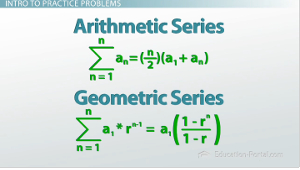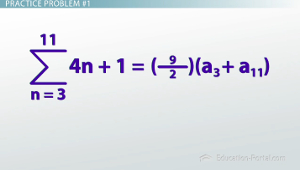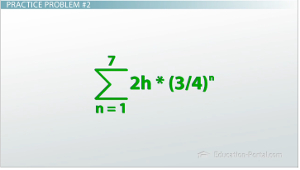# Arithmetic and Geometric Series: Practice Problems

An error occurred trying to load this video.

Try refreshing the page, or contact customer support.

Coming up next: Practice Problem Set for Sequences and Series

### You're on a roll. Keep up the good work!

Replay
Your next lesson will play in 10 seconds
• 0:47 Problem #1
• 3:26 Problem #2
• 5:51 Problem #3
• 7:48 Problem #4
• 10:06 Lesson Summary
Save Save

Want to watch this again later?

Timeline
Autoplay
Autoplay
Speed Speed

#### Recommended Lessons and Courses for You

Lesson Transcript
Instructor: Erin Monagan

Erin has been writing and editing for several years and has a master's degree in fiction writing.

There are many different types of problems concerning series and sequences - including some that are pretty abstract. From bouncing balls to adding up odd numbers, test your skills here with additional arithmetic and geometric series practice!

## Intro to Practice Problems

Now that you're familiar with both arithmetic and geometric series, it's time to test your skills with a few more examples. We'll need to remember the two shortcuts for finding arithmetic and geometric series. These two formulas are all we need for these examples. With that being said, these problems are a little more abstract than ones we've already done in previous lessons, so you're going to have to do some thinking before you can plug in numbers and be done.Because of that, it may be a good idea to pause the video after I've finished each example (maybe rewind it to the beginning), write the problem down and try to do it yourself without me helping. This way, you'll quiz yourself to make sure you understand what each question is asking you before you go on to the next one.

## Practice Problem #1

So, let's get take a look at our first example. I see a sigma, which tells me I'm taking a sum, and then the rule (directly to the right of that) looks like y = mx + b. So, I know it's an arithmetic sum, which means I'm going to be using this formula: sigma = (n/2)(a_1+a_n). But, there is once difference to this problem that we haven't looked at, and that is that the sum does not start at n = 1, it starts at n = 3.

That's going to change two things about how we use the formula to solve this problem. First off, in the formula, a_1 is the first term in the series, but in this case, I'm not substituting n = 1 for my first term, I'm substituting n = 3. In this problem, we're going to substitute a_1 with a_3 because a_3 is the first term in my series.

Second of all, the n in the n/2 part of this formula, represents the number of terms in the series. In this case, it's not as easy as substituting n_11. Since I'm starting at 3 and going up through 11, there aren't actually 11 different entries in this series. Because I'm starting at 3, I'm skipping over number 1 and 2. So, there are actually only 11 - 2 terms, which is 9 terms in this series.

A common mistake is to do 11 - 3 = 8 (so the top number minus the bottom number and that would give you 8), but that would be incorrect because it would imply that we are excluding 3 (because we'd be subtracting 3 away). We want to include 3, so we should do 11 - 2 = 9, because only the first and second entries are the ones excluded. That's how we get 9.Now that we've substituted all the correct values, it's easy as evaluating the formula out. So, a_3 we find by substituting 3 for our formula 4n + 1. And, a_n is our last term, so in this case we'll substitute 11 into the formula and we end up with: (9/2) * ((4(3) + 1) + (4(11) + 1)). Now, do the problem and multiply by 4.5 and you find the sum is 261.

## Practice Problem #2

Our second example is a very common word problem that goes like this: a ball is dropped from an unknown height (h) and it repeatedly bounces on the floor. After each bounce, the ball reaches a height that is 3/4 of the height from which it previously fell. How could we represent the distance the ball bounces between the first and eighth bounces with sigma notation?

There are a few things to notice here. First is that this is a geometric sequence, because we find each new height by multiplying the previous one by 3/4.

Second, when the ball starts bouncing, not only will it be bouncing up, but it will also be bouncing down. It makes two trips for all its different bounces.

Lastly, we are only asked for the distance it goes between the first and eighth bounces. That means we don't want to include the initial drop or the eighth bounce.

So, now we know all the information that we should need. We can begin writing our expression. Let's start with the rule that represents the situation. It's a geometric sequence so it's (a_1) * (r_n - 1) = a_1((1 - r_n) / (1 - r)). My a_1 (the initial starting point) is h because that's where we drop it from, and my common ration r is 3/4 because it goes 3/4 as high with each bounce.Now, I can add in the sigma notation in front of the rule and in this case we want to go from the first to the eighth bounce. So, I'll put n = 1 on the bottom and 8 on the top. We need to take into account that we don't want to include the initial drop. We could put n = 2 on the bottom (which is a fancier way you should be aware of). Instead of writing n = 2, we could change the exponent to just an n instead of n - 1. Doing that would do the same thing as if we put n = 2 in here.

To unlock this lesson you must be a Study.com Member.

### Register to view this lesson

Are you a student or a teacher?

#### See for yourself why 30 million people use Study.com

##### Become a Study.com member and start learning now.
Back
What teachers are saying about Study.com

### Earning College Credit

Did you know… We have over 200 college courses that prepare you to earn credit by exam that is accepted by over 1,500 colleges and universities. You can test out of the first two years of college and save thousands off your degree. Anyone can earn credit-by-exam regardless of age or education level.